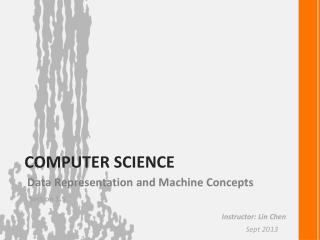Download PresentationComputer SCIENCE

# Computer SCIENCE - PowerPoint PPT PresentationDownload Presentation## Computer SCIENCE

- - - - - - - - - - - - - - - - - - - - - - - - - - - E N D - - - - - - - - - - - - - - - - - - - - - - - - - - -
##### Presentation Transcript

1. Data Representation and Machine Concepts Computer SCIENCE Section 1.5 Instructor: Lin Chen Sept 2013

2. 1 Data Storage 1.5 The Binary System Binary Notation Binary Addition Fractions in Binary Pearson Custom: Computer Science, Data Representation and Machine Concepts

3. 1 Data Storage Binary Notation Recall How to convert binary notation to decimal notation How to convert decimal notation to binary notation Convert 11010012 to decimal notation Convert 12310 to binary notation

4. 1 Data Storage Binary Addition Binary addition rule 0 + 0 = 0 1 + 0 = 1 0 + 1 = 1 1 + 1 = 10 1 + 1 + 1 = 11 10010011 + 111011

5. 1 Data Storage Binary Addition Binary addition rule 0 + 0 = 0 1 + 0 = 1 0 + 1 = 1 1 + 1 = 10 1 + 1 + 1 = 11 1 10010011 + 111011 0

6. 1 Data Storage Binary Addition Binary addition rule 0 + 0 = 0 1 + 0 = 1 0 + 1 = 1 1 + 1 = 10 1 + 1 + 1 = 11 11 10010011 + 111011 10

7. 1 Data Storage Binary Addition Binary addition rule 0 + 0 = 0 1 + 0 = 1 0 + 1 = 1 1 + 1 = 10 1 + 1 + 1 = 11 11 10010011 + 111011 110

8. 1 Data Storage Binary Addition Binary addition rule 0 + 0 = 0 1 + 0 = 1 0 + 1 = 1 1 + 1 = 10 1 + 1 + 1 = 11 11 10010011 + 111011 1110

9. 1 Data Storage Binary Addition Binary addition rule 0 + 0 = 0 1 + 0 = 1 0 + 1 = 1 1 + 1 = 10 1 + 1 + 1 = 11 1 11 10010011 + 111011 01110

10. 1 Data Storage Binary Addition Binary addition rule 0 + 0 = 0 1 + 0 = 1 0 + 1 = 1 1 + 1 = 10 1 + 1 + 1 = 11 11 11 10010011 + 111011 001110

11. 1 Data Storage Binary Addition Binary addition rule 0 + 0 = 0 1 + 0 = 1 0 + 1 = 1 1 + 1 = 10 1 + 1 + 1 = 11 11 11 10010011 + 111011 1001110

12. 1 Data Storage Binary Addition Binary addition rule 0 + 0 = 0 1 + 0 = 1 0 + 1 = 1 1 + 1 = 10 1 + 1 + 1 = 11 11 11 10010011 + 111011 11001110

13. 1 Data Storage Binary Addition Questions: 1001001 + 0011001 1000111 + 0010110 http://www.atilim.edu.tr/~compe111/

14. 1 Data Storage Fractions in Binary Convert fractional binary number to decimal number 1 0 -1 -2 -3 • 0 . 0 1 1 = 1* 21 + 0*20 + 0*2-1+ 1*2-2+ 1*2-3 • = 2 + 0 + 0 + + • = 2.375 Questions: Convert following two base notations to their equivalent ten base notation 100.110 111.001 0.101

15. 1 Data Storage Fractions in Binary Convert fractional decimal number to binary number 2.375 Integer Part Fractional Part 0 210 = 102 0.375 * 2 = 0.75 1 0.75 * 2 = 1.5 1 0.5 * 2 = 1.0 10.011

16. 1 Data Storage Fractions in Binary Questions: Convert following fractional decimal number to binary number 10.1 4.5 1.125